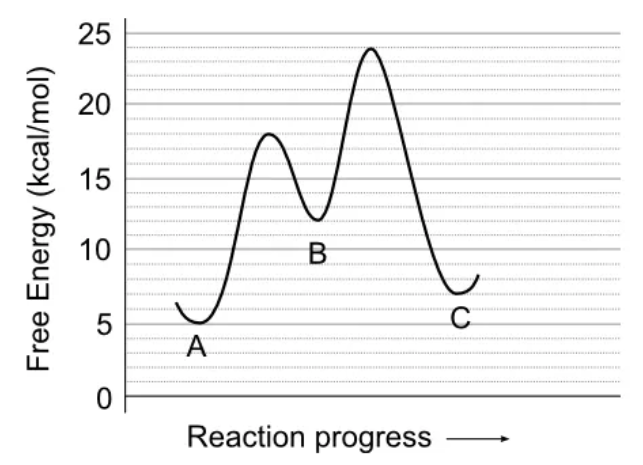# Problem: Use the reaction energy diagram above to answer the following questions. Calculate the activation energy, ΔG for the step C to B _____ kcal/mol Calculate the overall energy change, ΔG°, for the process B to A. ________kcal/mol. Which step is faster, (a) B to A or (b) B to C?

###### FREE Expert Solution

Activation energy  Highest peak - Starting Energy

For step B to C, step is at 12 kcal/mol while the peak is at 24 kcal/mol

24 - 12 = 12 kcal/mol

91% (102 ratings)###### Problem DetailsUse the reaction energy diagram above to answer the following questions.

Calculate the activation energy, ΔG for the step C to B _____ kcal/mol

Calculate the overall energy change, ΔG°, for the process B to A. ________kcal/mol.

Which step is faster, (a) B to A or (b) B to C?